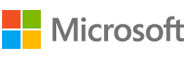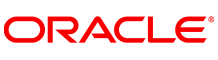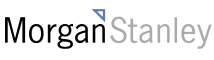New update is available. Click here to update.
Topics

# First Missing Positive

Moderate0/80
Average time to solve is 18m+34 more companies

## Problem statement

You are given an array 'ARR' of integers of length N. Your task is to find the first missing positive integer in linear time and constant space. In other words, find the lowest positive integer that does not exist in the array. The array can have negative numbers as well.

For example, the input [3, 4, -1, 1] should give output 2 because it is the smallest positive number that is missing in the input array.

Detailed explanation ( Input/output format, Notes, Images )
Sample Input 1 :
``````1
5
3 2 -6 1 0
``````
Sample Output 1:
``````4
``````
Explanation for Input 1:
``````The first positive number is 1 and it is present in the array similarly 2 and 3 are also present in the array. 4 is missing from the array. Thus, the minimum positive integer that is missing is 4.
``````
Sample Input 2 :
``````1
5
0 1 2 3 4
``````
Sample Output 2:
``````5
``````Console